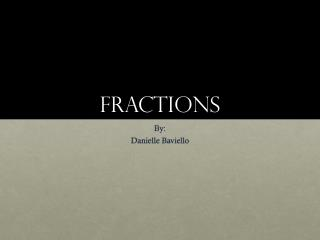# Fractions - PowerPoint PPT PresentationDownload PresentationFractions

Fractions
Download Presentation## Fractions

- - - - - - - - - - - - - - - - - - - - - - - - - - - E N D - - - - - - - - - - - - - - - - - - - - - - - - - - -
##### Presentation Transcript

1. Fractions By: Danielle Baviello

2. Objectives • Students will be able to describe in writing the definitions of fraction, numerator, and denominator. • Students will be able to pronounce three (3) examples of fractions given in this presentation. Examples would be: 1/10 is “One Tenth”. • Students will be able to show the teacher specific fractions using representative pieces of pizza. • Students will be able to simplify fractions to 1/2 on paper when asked.

3. What is a fraction? • A fraction: names part of a region or part of a group.

4. Fraction definitions • Numerator is: the top number of a fraction (part) • Denominator is: the bottom number of a fraction (whole) number of partnumerator _____________________ number of wholedenominator

5. Examples of Pizza There are 8 slices in a pizza pie. How many parts of the whole have been eaten in each example? Numerator: 6 Numerator: 4 Numerator: 7 Numerator: 2 ---- ---- ---- ---- Demoninator: 8 Denominator: 8 Denominator: 8 Denominator: 8

6. More Fractions and how they are pronounced “One whole” “One half” “One third” “One fourth” “One fifth” “One sixth” “One seventh” “One eighth” “One ninth” “One tenth” “One eleventh” “One twelfth” Other numbers would be pronounced the same as the numerator. 2/3 is said “two thirds” or “two out of three.”

7. Simplifying Fractions • Some fractions can be simplified into smaller numbers to be easier to understand. An example would be the 4/8 fraction that we saw earlier in the Examples of Pizza. • This example could be simplified from 4/8 to 1/2. This picture shows that 4 out of 8 of the pizza slices are gone and that is also half of the total. We can see that half of the pizza is gone.

8. Simplifying Continued • Other fractions could be simplified to 1/2 • A fraction like 40/80 can also be simplified to 1/2. We know that 40 is half of 80 so it makes sense that this would be simplified to 1/2. • You can also see if the fraction would be simplified to 1/2 by seeing how many times 40 goes into 80. 1 40 goes into 80 2 times and that’s how we get 1/2. • This also works with fractions like 15/30.

9. Review What is a fraction? What is the top of a fraction or part of the whole? What is the bottom of a fraction or the whole itself? How would you say 4/5, 6/8, 100/2000? How would you write “three fourths,” “eight tenths,” “nine thirteenths?”

10. Review Continued.. • Simplify 4/8 • Simplify 40/80 • Simplify 50/100 • Simplify 15/30

11. Class activity • For a fun class activity the teacher will supply each students will a small bag of M&M’s and a sheet to go along with it. • The sheet will ask the students to calculate what fraction of their bag is red, blue, green and yellow. • Each students will have a different amount of candy and a different amount of each color. • When the students complete their sheet they will share with the students next to them. The students can show their classmates, using the candy, how they got each fraction on their sheet.

12. Online assessment • https://testmoz.com/95253